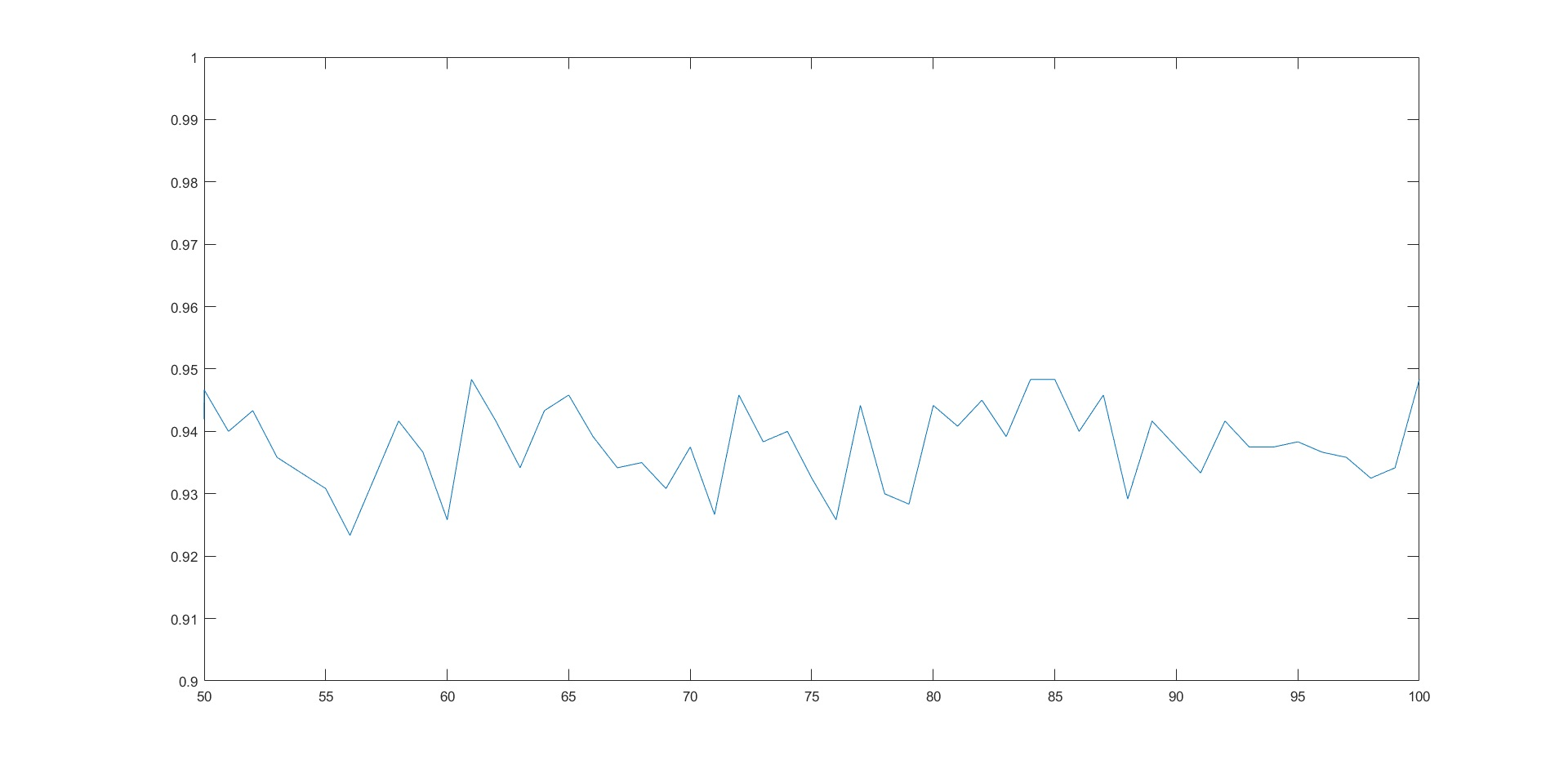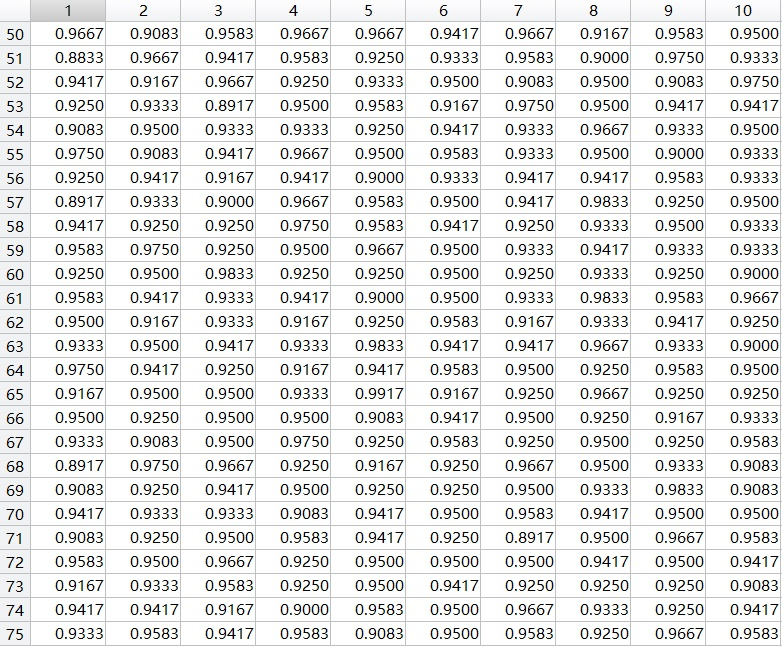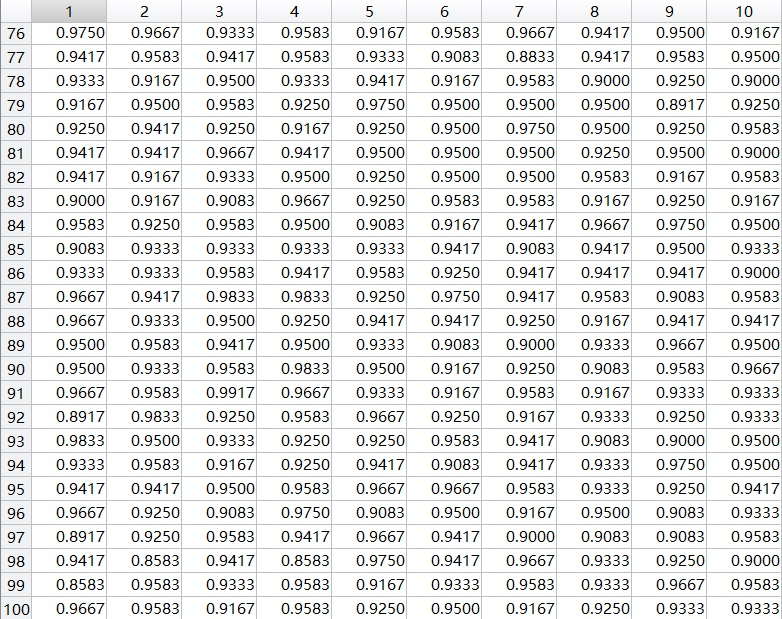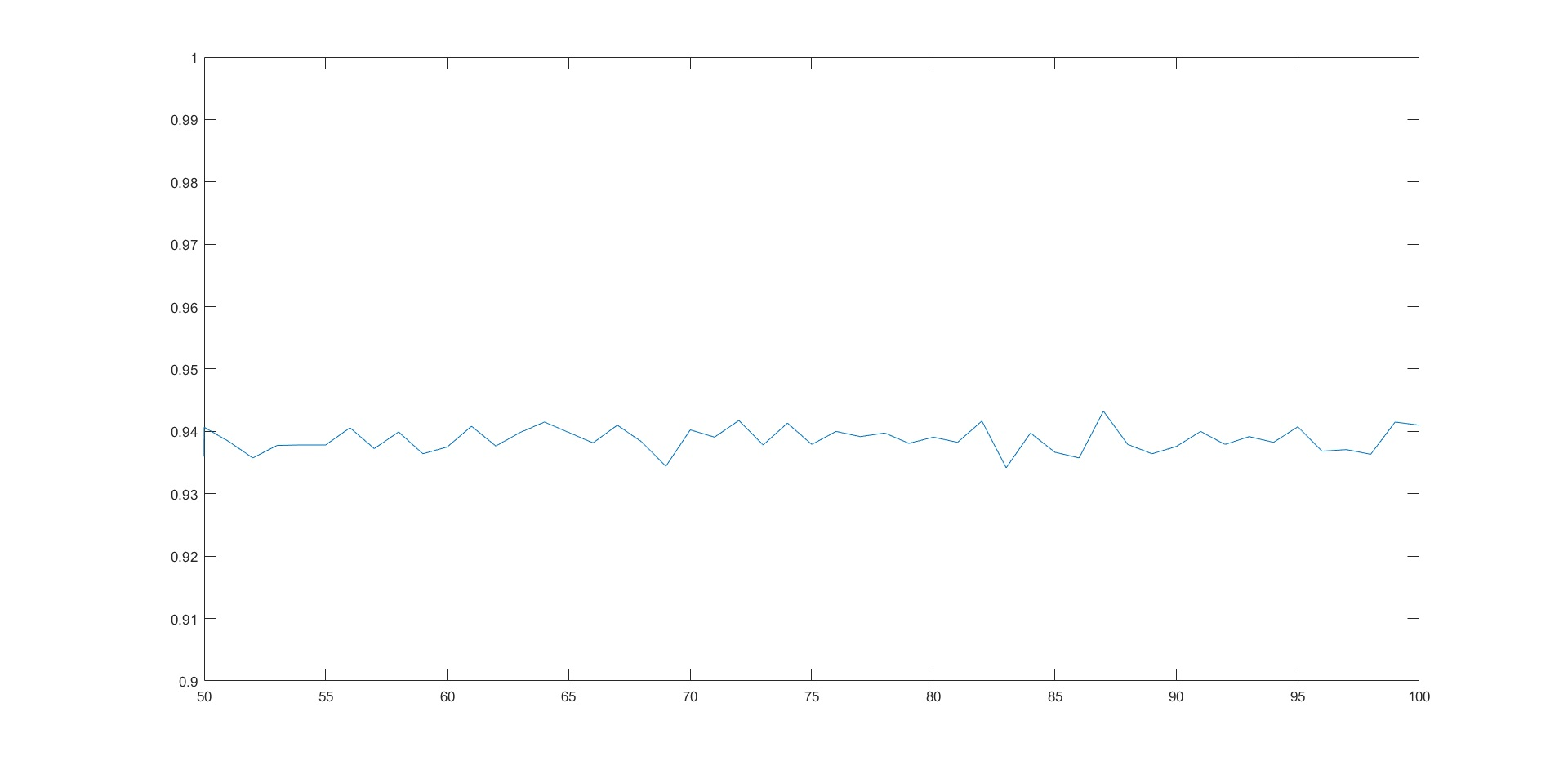# 基于PCA的人脸识别

## 一、算法描述

1.首先，应题目要求，对于所有的Faces集里的40个人，各随机取出其10张图像中的7张用作训练集，剩余3张用作后续的测试。

2.然后，将280张train_imgs都拉伸成列向量并将所有列拼在一起，由于每张图像的总像素数都为10304，这样就得到了10304*280的矩阵X。

3.X的每列再减去均值向量，从而中心化。

4.求出X的转置和X的矩阵乘积，并求出乘积40*40矩阵的特征向量，这里用的是matlab的eig函数。

5.滤出前K大的特征值对应的特征向量W，再将X乘上W映射得到V，将V的每一列向量作为后续映射关系的一组基向量，共有K个基向量，也可以称为K个特征脸。

6.将X每一列都通过基向量矩阵V映射到对应的特征空间中。这样相当于将每张图像train_imgs都在新的空间中找到了对应的位置。

7.对于每个测试图像，也进行类似上述的变换：转成列向量，减去均值向量而中心化，然后用基向量矩阵映射到特征空间中。

8.要判断测试图像和40张train_imgs的哪张最匹配，只需对比测试图像在特征空间的新坐标和40张train_imgs在特征空间的坐标直接的欧几里得距离（或二范数）的大小，找到二范数最小的对应的train_img，就找到了最匹配训练图像了。

9.综上所述，这种算法的主要思想就是，去除部分无关的或者关系较小的向量，保留影响较大的向量作基，这样即减少了基向量的数目从而减少了运算量，同时又减少了图像细节，能避免无关的向量和测试图像主人公的表情、脸朝向和配饰等变化对测试准确性产生不良干扰。

## 二.Matlab代码

function [ imgs ] = Get_Training_Set( input_path, index, height, width, output_path )
imgs = zeros(length(index), height, width);
for i = 1 : length(index)
imgs(i, :, :) = uint8(imread([input_path '/' num2str(index(i)) '.pgm']));
end
end

function [ found ] = Test_Case( V, eigenfaces, indexes, i, j, mean_img )
f = imread(['Faces/S' num2str(i) '/' num2str(indexes(i, j)) '.pgm']);
[height, width] = size(f);
f = double(reshape(f, [height * width, 1])) - mean_img;
f = V' * f;
[~, N] = size(eigenfaces);
distance = Inf;
found = 0;
for k = 1 : N
d = norm(double(f) - eigenfaces(:, k), 2);
if distance > d
found = k;
distance = d;
end
end
end

N = 7 * 40; K = 90;
Test_Num = 3 * 40;
height = 112;
width = 92;
Test_Times = 100;
accuracy = zeros(100, Test_Times);
for K = 50 : 100
for T = 1 : Test_Times
indexes = zeros(N, 10);
train_imgs = zeros(N, height, width);
for i = 1 : 40
indexes(i, :) = randperm(10);
train_imgs((i - 1) * 7 + 1 : i * 7, :, :) = Get_Training_Set(['Faces/S' num2
end
train_imgs = uint8(train_imgs);
X = zeros(height * width, N);
for i = 1 : N
X(:, i) = reshape(train_imgs(i, :, :), [height * width, 1]);
end
mean_img = mean(X, 2);
for i = 1: N
X(:, i) = X(:, i) - mean_img;
end
L = X' * X;
[W, D] = eig(L);
W = W(:, N - K + 1 : N);
V = X * W;
eigenfaces = V' * X;
for i = 1 : 40
for j = 8 : 10
found = Test_Case(V, eigenfaces, indexes, i, j, mean_img);
found = floor((found - 1) / 7) + 1;
if found == i
accuracy(K, T) = accuracy(K, T) + 1;
end
end
end
accuracy(K, T) = double(accuracy(K, T) / (Test_Num));
end
end
Mean_Accuracy = mean(accuracy, 2);
plot(Mean_Accuracy); axis([50 100 0.9 1]); 

## 三、测试性能表格

found = Test_Case(V, eigenfaces, indexes, i, j, mean_img);
found = floor((found - 1) / 7) + 1;## 四、过程及结果图像